# Poisson formula for harmonic functions

Jump to: navigation, search

Consider a harmonic function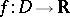defined in a domainin a Euclidean space,. Let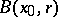denote the open ball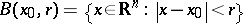with centreand radius. Assume that the closure of this ball is contained in.

The classical Poisson formula expresses thatcan be recovered inside the ball by the values ofon the boundary of the ball integrated against the Poisson kernelfor the ball,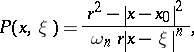(a1)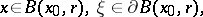where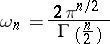is the-dimensional surface area of the unit ball in.

The Poisson formula is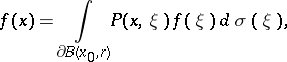whereis the surface measure of the ball, the total mass of which is.

For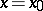the formula reduces to the mean-value theorem for harmonic functions, stating that the value at the centre of the ball is the average over the boundary of the ball.

The same type of formula holds when the ball is replaced by a bounded domainwith a sufficiently smooth boundary and such that the closure ofis contained in. The Poisson kernel (a1) is replaced by the Poisson kernel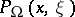forandis replaced by the surface measure on the boundary of. The Poisson kernel, defined on, is given as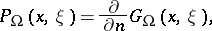where the inward normal derivative of the Green function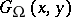forwith respect to the second variableis used.

For each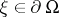the function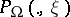is positive and harmonic in, and for each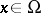the measure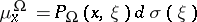is a probability, called the harmonic measure forat.

The Poisson kernel has the properties ()and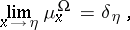where the last limit is in the weak topology for probability measures on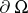(cf. also Weak convergence of probability measures) andis the Dirac distribution at.

There are only a few cases where the Poisson kernel can be given in closed form as for the ball.

The Poisson formula for a domainis related to the solution of the Dirichlet problem: For a function, the harmonic continuation inis (under suitable assumptions) given asThere is also a Poisson formula for unbounded domains, the simplest of which is for the upper half-spaceThe formula is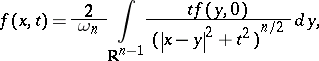and it is valid for a harmonic function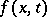in the upper half-space provided it has a continuous extension to the closure and satisfies some growth condition. See Hardy spaces.